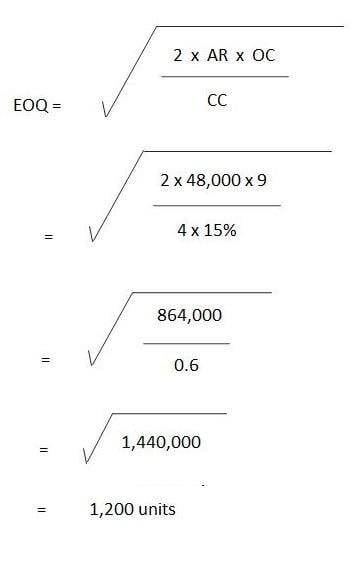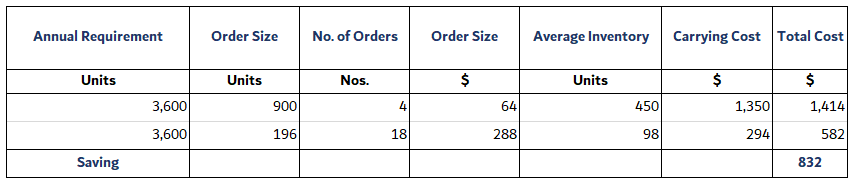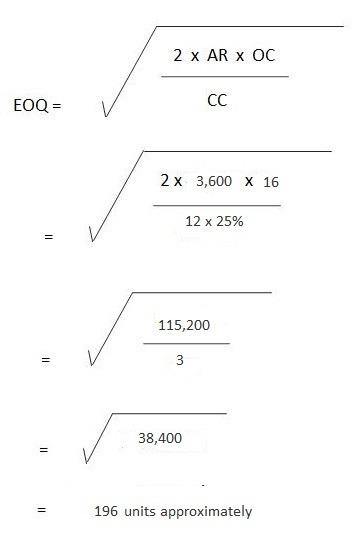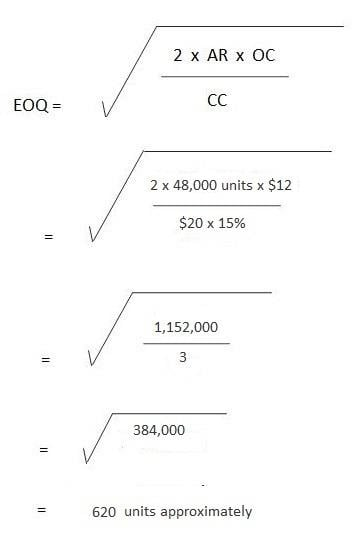## Problem 1

The John Equipment Company estimates its carrying cost at 15% and its ordering cost at \$9 per order. The estimated annual requirement is 48,000 units at a price of \$4 per unit.

Required:

1. What is the most economical number of units to order?
2. How many orders should be placed in a year?
3. How often should an order be placed?

### Solution

1. What is the most economical number of units to order?

Annual requirement = 48,000 units
Ordering cost = \$9 per order
Carrying cost = 15% of per-unit cost
Per unit cost = \$4 per unit2. How many orders should be placed in a year?

= Annual requirement / EOQ
= 48,000 units / 1,200 units
= 40 orders

3. How often should an order be placed?

Frequency of orders = No. of days in one year / No. of orders
= 360 days / 40 orders
= 9 days

## Problem 2

To date, Raymond Bro. has been purchasing an item in lots of 900 units. This equates to a three-month supply. The cost per unit is \$12, the order cost is \$16 per order, and the carrying cost is 25%.

Required: How much can Raymond Bro. save per year by purchasing the item in the most economical quantities?

### SolutionThe first stage in our working is to compute the annual requirement.

Given that 900 units amounts to a three-month supply, the monthly requirement is 900 units / 3 months = 300 units.

Therefore, the annual requirement is 300 units x 12 months = 3,600 units.

In turn, the EOQ can be computed as follows:No. of Orders = 3,600 units / 900 units
= 4 orders
= 3,600 units / 196 units
= 18 orders approx.
Ordering Cost = 4 orders x \$16 per order
= \$64
Also, in the case of EOQ:
= 18 orders x \$16 per order
= \$288
Average Inventory = 900 units / 2
= 450 units
In the case of EOQ:
= 196 units / 2
= 98 units
Carrying cost = \$3 x 450 units
= \$1,350
In the case of EOQ:
= \$3 x 98 units
= \$294
Total cost = \$64 + \$1,350
\$1,414
In the case of EOQ:
=\$288 + \$294
= \$582
Saving = \$1,414 – \$582
= \$832

## Problem 3

A manufacturing company places a semi-annual order of 24,000 units at a price of \$20 per unit. Its carrying cost is 15% and the order cost is \$12 per order.

Required:

1. What is the most economical order quantity?
2. How many orders need to be placed?

### SolutionNo. of orders per year = Annual Requirement / EOQ
= 48,000 units / 620 units
= 77 orders approximately

To compute the annual requirement:

24,000 units are ordered semiannually, therefore:
Annual requirement = 24,000 units x 2 = 48,000 units.

### Keep Learning

What a brain exercise that was! Interested to know more about this topic? We’re happy to connect you to a financial advisor in Lake Mary, FL. But if you live outside the locale, fret not. Check on our financial advisor page to learn which areas we are currently working with.

### What is the Economic Order Quantity (EOQ) formula?

EOQ stands for economic order quantity. The formula is: EOQ = √ (2xARxOC) / CC where AR = annual requirements, OC = per unit cost, and CC = carrying cost per unit per year of materials inventory

### What does “efficient” mean? Explain why it is so helpful in business.

Efficient means to use the minimum resources to achieve a goal. In this case, it means minimizing your inventory amount. It is helpful because it decreases the money you spend on holding inventory, and lowers the risk of not having enough inventory if a sudden increase was to happen.

### What are some examples of Economic Order Quantity (EOQ) applications in real life?

Clothing stores order a certain amount in inventory at a time to save on costs. A clothing store, for example, has an EOQ of 10 and sales are going well. If the owner wanted to make more money or generate more sales, they would increase that EOQ amount so they won’t have to keep placing orders as often, saving them time and money in the process.

### What are the advantages of calculating Economic Order Quantity (EOQ)?

It minimizes carrying costs and saves time. You can also save a substantial amount of money using this method since you would not have to place as many orders reducing supplier lead time

### What are the disadvantages of calculating Economic Order Quantity (EOQ)?

EOQ does not account for seasonal or economic fluctuations which can cause huge differences in your inventory levels. It does not take into account the random nature of demand.

True is a Certified Educator in Personal Finance (CEPF®), a member of the Society for Advancing Business Editing and Writing, contributes to his financial education site, Finance Strategists, and has spoken to various financial communities such as the CFA Institute, as well as university students like his Alma mater, Biola University, where he received a bachelor of science in business and data analytics.

To learn more about True, visit his personal website, view his author profile on Amazon, his interview on CBS, or check out his speaker profile on the CFA Institute website.